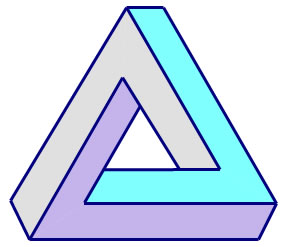"Where there is matter, there is geometry."
- Johannes Kepler

# Geometry## Handbooks

Students of Geometry may find the following Handbooks useful as references.

## PC Apps

There is no specific Geometry App, but when the student begins to study basic Trigonometry, the Trigonometry App may be of interest.

## Euler Line Challenge

Here's a very cool problem that has been around for a very long time. Prove that the Centroid (intersection of medians), Orthocenter (intersection of altitudes) and Circumcenter (intersection of perpendicular bisectors) of any triangle lie on the same line. (Note: this line is called the Euler Line.)

Try to prove this yourself. One solution is provided below.

## Sample Problems

The value of reviewing these sample problems and solutions is twofold. The more obvious benefit relates to problems that a student has difficulty solving themselves; a solution is provided. Less obvious is the set of techniques used in our solutions; some of the techniques used on the sample problems may not be taught in the classroom. We concentrate on efficient solutions and shortcuts; so a review of the solved problems may present new techniques that enable a student to be more efficient in their approach to solving problems.